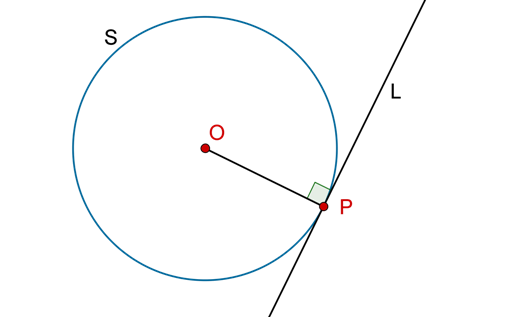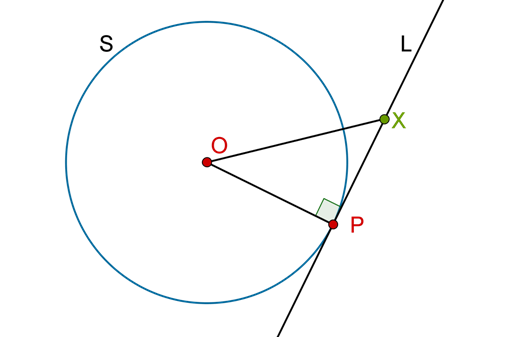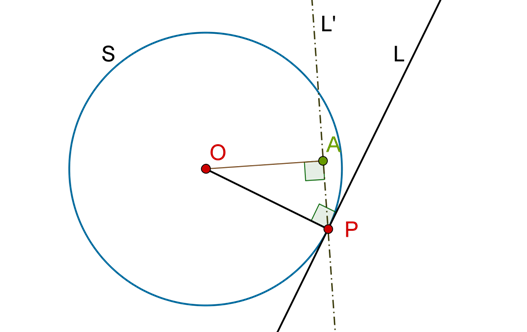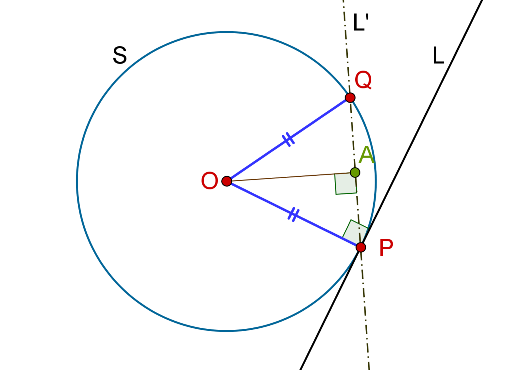# Uniqueness of the Tangent at a Point

Uniqueness of the Tangent at a Point

Theorem. At any point on a circle’s circumference, one and only one tangent can be drawn to it, and this tangent is perpendicular to the radius through the point of contact.

This theorem has two parts, and we will prove it in that sequence:

Part 1: We will take a point P on the circumference of a circle S with center O. Draw a line L perpendicular to OP:We will prove that L is a tangent to S.

Part 2: Then, we will prove that L is unique, that is, no other tangent through P can be drawn to S.

Proof (Part-1): Note that OP is the radius through P, and $$OP \bot L$$ by our construction. Now, if you take any point X on L other than P, we must have:

$OX{\rm{ }} > {\rm{ }}OP$

This is because the shortest distance of a point from a line is along the perpendicular. Consider the following figure:Clearly, OX is the hypotenuse in the right triangle OXP, and hence $$OX{\rm{ }} > {\rm{ }}OP$$. But since OP is the radius, this means that X lies outside S. This is true for every point on L, other than P. Thus, L intersects S in only one point (P), and hence L is a tangent to S.

Proof (Part-2): Suppose that a line different from L, say L’, is drawn through P. If L’ passes through the circle’s center, then it will obviously intersect the circle again, at a point different from P (and will therefore not be a tangent).

Suppose that L’ does not pass through O. We now need to show that it will not be a tangent to S in any case, that is, it will intersect S again at a point different from P. Draw OA, the perpendicular from O onto L’ (note that A will be different from P, as L’ is not perpendicular to OP):Now, we simply take a point Q on L’ such that PO = OQ (this can always be done). Thus, the point Q must also lie on the same circle S (why?), which means that L’ intersects S at Q (apart from P), and is therefore not a tangent to it: